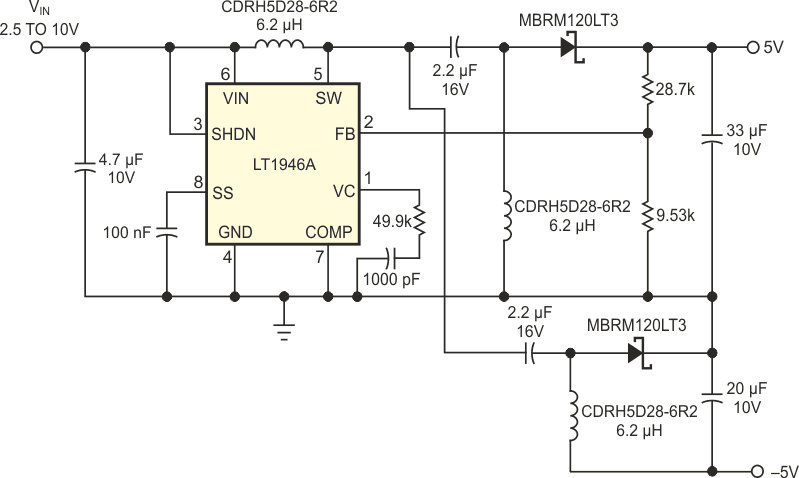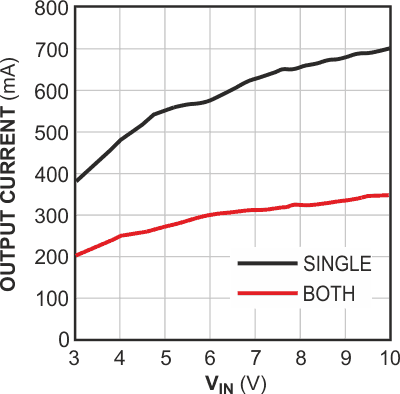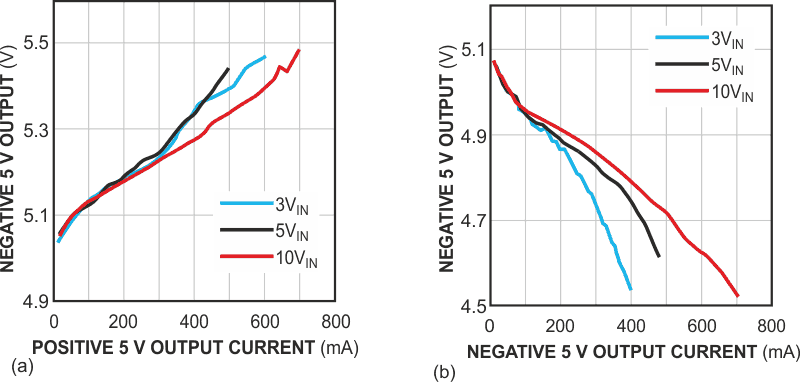# Transformerless dc/dc converter produces bipolar outputs

## Linear Technology LT1946A

The conventional way to produce dual (positive and negative) outputs from a single positive input is to use a transformer. Although such designs are relatively simple, the transformer inherently introduces the problem of size. It can be challenging to fit a transformer into an application in which it's important to minimize the circuit footprint and height. The circuit in Figure 1 generates ±5 V from a 3 to 10 V input and fits into applications that lack the room to accommodate a transformer. The circuit uses a topology that allows the disconnection of both outputs when the dc/dc converter is in shutdown mode; thus, the quiescent current is low during shutdown (standby) mode. The circuit also produces a regulated positive and negative 5 V, regardless of whether the input is higher or lower than 5 V. Therefore, the circuit can operate from various input sources, such as a  3 to 4.2 V lithium-ion battery or a 3.3 to 10 V wall adapter. By slightly modifying the circuit, you can increase the input range to 2.5 to 16 V and the output range to 3 to 12 V.Figure 1. A simple circuit produces –5 V from a single positive input without the need for a transformer.

The 2.7-MHz switching frequency of the dc/dc converter allows the use of small, low-profile external components (input/output capacitors and inductors). Using three small inductors instead of one bulky transformer not only reduces the size and height of the converter, but also evenly distributes the power dissipation over the board, thus eliminating concentrated hot spots. The output-current capability of the circuit increases as the input voltage increases (higher input voltage, lower input current). Figure 2 shows the maximum output current versus the input voltage. The “both” curve represents the maximum allowable output current of both ±5 V outputs when you load them with the same current. The “single” curve represents the maximum allowable output current of each output when you load either output alone. When the current from one output decreases, the current capability of the other output increases, as long as you do not exceed the current rating of the dc/dc converter.Figure 2. This graphic shows maximum output current versus input voltage for both outputs or a single output.

Cross-load regulation is another important design consideration in this type of circuit. Because the –5 V output does not have control of the dc/dc converter's PWM feedback, the –5 V output voltage changes with output current. You can greatly improve the cross-load regulation by adding a 10- to 20-mA preload at each output. The preload ensures that the dc/dc converter operates in continuous-conduction mode, in which the inductor current is stable enough to provide constant current. Figure 3 shows the –5 V output voltage regulation under different load conditions at the positive (Figure 3a) and negative (Figure 3b) outputs. In this case, to improve cross-load regulation, both the outputs connect to a 20-mA preload.Figure 3. These curves show the regulation of the –5 V supply as a function of the 5 V output current (a), and the regulation of the same output as a function of the –5 V output current (b).

## Materials on the topic

EDN

You may have to register before you can post comments and get full access to forum.
 User Name Remember Me? Password